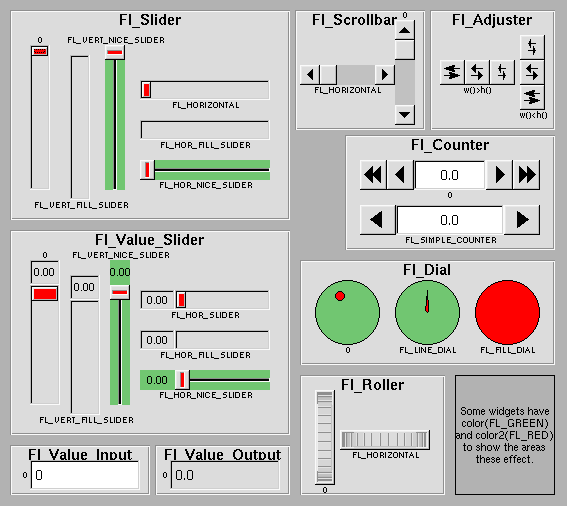# NAME

Fl::Valuator - Controls a Single Floating-Point Value

# Description

The Fl::Valuator controls a single floating-point value and provides a consistent interface to set the value, range, and step, and insures that callbacks are done the same for every object.

There are probably more of these classes in FLTK than any others:In the above diagram each box surrounds an actual subclass. These are further differentiated by setting the C of the widget to the symbolic value labeling the widget. The ones labelled "0" are the default versions with a C. For consistency the symbol FL_VERTICAL is defined as zero.

# Methods

Fl::Valuator inherits from Fl::Widget. On top of that, it exposes the following methods...

## `bounds(...)`

``    \$val->bounds(0, 100);``

Set the minimum and maximum values for the valuator widget.

## `clamp(...)`

``    my \$in_range = \$val->clamp(500);``

Clamps the passed value to the valuator range.

## `maximum(...)`

``    \$val->maximum(100);``

Set the maximum value for the valuator widget.

``    my \$max = \$val->maximum( );``

Get the maximum value for the valuator widget.

## `minimum(...)`

``    \$val->minimum(0);``

Set the minimum value for the valuator widget.

``    my \$min = \$val->minimum( );``

Get the minimum value for the valuator widget.

## `precision(...)`

``    \$val->precision(3);``

Set the step value to `1/10^digits`.

## `range(...)`

``    \$val->range(20, 50);``

Sets the minimum and maximum values for the valuator.

When the user manipulates the widget, the value is limited to this range. This clamping is done after rounding to the step value (this makes a difference if the range is not a multiple of the step).

The minimum may be greater than the maximum. This has the effect of "reversing" the object so the larger values are in the opposite direction. This also switches which end of the filled sliders is filled.

Some widgets consider this a "soft" range. This means they will stop at the range, but if the user releases and grabs the control again and tries to move it further, it is allowed.

The range may affect the display. You must `redraw()` the widget after changing the range.

## `round(...)`

``    my \$value = \$val->round(50.45);``

Round the passed value to the nearest step increment.

Does nothing if step is zero.

## `step(...)`

``    my \$value = \$val->step(50.45);``

Gets or sets the step value.

As the user moves the mouse, the value is rounded to the nearest multiple of the step value. This is done before clamping it to the range. For most widgets the default step is zero.

For precision the step is stored as the ratio of two integers, A/B. You can set these integers directly. Currently setting a floating point value sets the nearest A/1 or 1/B value possible.

## `value(...)`

``    \$val->(155);``

Sets the current value.

The new value is not clamped or otherwise changed before storing it. Use `clamp()` or `round()` to modify the value before calling `value()`. The widget is redrawn if the new value is different than the current one. The initial value is zero.

`changed()` will return true if the user has moved the slider, but it will be turned off by `value(\$x)` and just before doing a callback (the callback can turn it back on if desired).

``    my \$value = \$val->value( );``

Gets the floating point value.